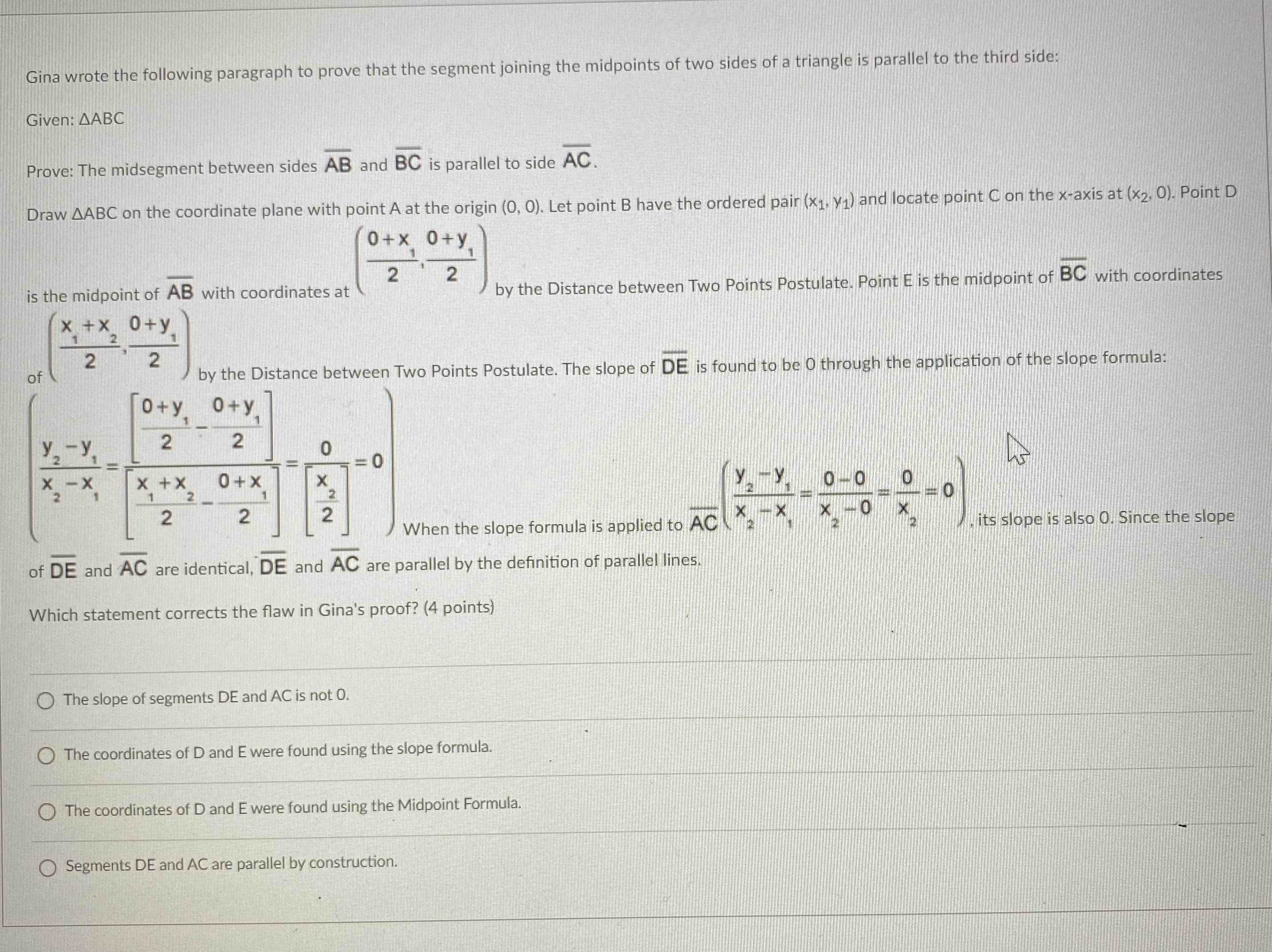### Still have math questions?

Trigonometry
QuestionGina wrote the following paragraph to prove that the segment joining the midpoints of two sides of a triangle is parallel to the third side: Given: $$\triangle ABC$$

Prove: The midsegment between sides $$\overline { AB }$$ and $$\overline { BC }$$ is parallel to side $$\overline { AC }$$ .